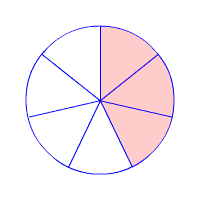Identifying Fractions
Plotting Fractions
Fraction Vocabulary
Fraction Equivalency (True or False)
100What is one half(1/2)?

100

Plot 1/2100

1/2 + 1/2

What is 1?

100

The numbers on the top and bottom of a fraction

What is the numerator and denominator?

100

1/2 = 4/6

What is false?

200What is three fourths (3/4)?

200

Plot 3/8200

1/6 + 3/6

What is 4/6?

200

The numerator and the denominator represents...

What is the total number of equal parts shaded or being considered and the total number of equal parts that make a whole?

200

2/3 = 4/6

What is true?

300What is five sixths (5/6)?

300

Plot 8/16300

1 1/5 + 1/5

What is 1 2/5?

300

Separating or breaking something into smaller pieces is called

What is partitioning?

300

7/14 = 1/3

What is false?

400What is 3/7?

400

Plot 1 5/6400

1 2/7 + 1 1/7

What is 2 3/7?

400

The name of a fraction that has a larger numerator than denominator

What is an improper fraction?

400

18/6 = 3

What is true?

500What is 5/5 or 1?

500

Plot 1 3/4500

2/6 + 1/3

What is 2/3 or 4/6?

500

The operation being performed in a fraction

What is division?

500

7/2 = 3

What is false?

Click to zoom